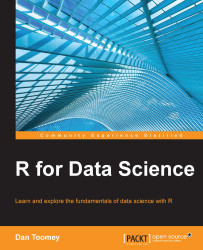•#### R for Data Science#### Overview of this book

R for Data ScienceCreditswww.PacktPub.comPrefaceFree Chapter
Data Mining PatternsData Mining SequencesText MiningData Analysis – Regression AnalysisData Analysis – CorrelationData Analysis – ClusteringData Visualization – R GraphicsData Visualization – PlottingData Visualization – 3DMachine Learning in ActionPredicting Events with Machine LearningSupervised and Unsupervised LearningIndex## K-means clustering

K-means is the process of assigning objects to groups so that the sum of the squares of the groups is minimized. R has the `kmeans` function available for cluster analysis. K-means is a method of determining clusters based on partitioning the data and assigning items in the dataset to the nearest cluster.

K-means clustering is done in R using the `kmeans` function. The `kmeans` function is defined as follows:

```kmeans(x, centers, iter.max = 10, nstart = 1,
algorithm = c("Hartigan-Wong", "Lloyd", "Forgy","MacQueen"), trace=FALSE)```

The various parameters of this function are described in the following table:

Parameter

Description

`x`

This is the dataset.

`centers`

This contains the number of centers/clusters to find.

`iter.max`

This stores the maximum number of iterations allowed.

`nstart`

This contains the number of random clusters to find.

`algorithm`

This contains the algorithm to be used to determine clusters. `Hartigan-Wong` is the default. `Lloyd` and `Forgy` are the same...